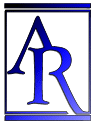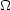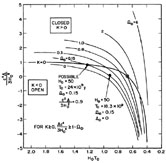Annu. Rev. Astron. Astrophys. 1988. 26: 561-630 Copyright © 1988 by Annual Reviews. All rights reserved

### 9. TIME-SCALE TEST

The value of the redshift-distance ratio (i.e. the Hubble constant) was first set at H0 = 558 ± 10 % km s-1 Mpc-1 (Hubble & Humason 1931). Using this determination it was soon evident that the redshift of the galaxies is a characteristic of the Universe itself, rather than being simply a local phenomenon. The evidence is the order-of-magnitude agreement between the expansion time scale associated with H0-1 (which is 1.7 × 109 yr for H0 = 558) and the age of the Earth, known even at the beginning of this century to be of order 109 yr [Holmes (1913), and many times thereafter following Boltwood (1907); cf. Holmes (1946, 1947, 1949) and Jeffreys (1924, 1948, 1949) for the early literature].

The conclusion was strengthened when the age of the oldest stars in the Galaxy was shown to be of the same order (Sandage & Schwarzschild 1952). This followed the earlier, several-decade debate on two time scales for the Galaxy. Bok (1946) reviewed the multiple evidence for the long and short time scale. Note from his review that even in 1946, evolution of stars was considered to be up rather than off the main sequence, giving no characteristic signal in the HR diagram by which to date the stars. This difficulty prevailed until Trumpler's (1925, 1928) discovery of main sequence termination points was theoretically understood in the early 1950s with the introduction of chemically inhomogeneous stellar models.

A third time scale was proposed by Rutherford (1929) that permitted age dating of the chemical elements. Ages of several times 109 yr for uranium was close enough to the Hubble time to be startling. In modem times the theme has been integrated into a general history of events that have spread the elements made in stars everywhere that there is matter (Hoyle 1946, 1947, 1954, Burbridge et al. 1957), setting the foundations for the study of the chemical evolution of galaxies.

Although order-of-magnitude agreement of the three cosmic clocks draws attention to the significance of the time-scale coincidence, a more detailed inquiry requires precision in the measurements. The standard model predicts the relation between the age of the Universe T0, the Hubble constant H0, and cosmological parameters q0 (or0) and. Many representations of this parameter space exist, such as that of Robertson (1955, 1963) for a specific value of H0; of Fowler (1987), who generalized Robertson's parametrization by using0 and H0T0 as axes; or of Stabell & Refsdal (1966, their Figure 5) in the density, q0 plane with H0T0 as parameter.

Figure 14 (from Sandage & Tammann 1986) is a diagram in the, H0T0,plane with0 as parameter, plotted from the tables of Refsdal et al. (1967) following Mattig (1958) and Solheim (1966). The well-known condition that T0 = 2/3H0-1 if0 = 1 and= 0 is marked by a crossed circle. Two other data points are marked by filled circles at values of H0 and T0 thought, in various past discussions, to be appropriate. The point to note about Figure 14 is that H0T0, which is in principle observable, permits0 to be determined ifis known (or assumed); conversely, it permitsto be found if H0T0 and0 are known. The special cut at= 0 in the diagram gives the0 = f (H0T0) relation calculated earlier (Sandage 1961a, Table 8).Figure 14. The time-scale test displayed in the H0T0,plane with0 as parameter. The flat space-time condition ofc2 = 3H03(1 -0) is shown by the heavy line marked K = 0. The special case of0 = 1,= 0, H0T0 = 2/3 is marked by a crossed circle (from Sandage & Tammann 1986).